# NCERT Solutions for Class 7 Maths Chapter 11 Perimeter and Area Ex 11.4

NCERT Solutions for Class 7 Maths Chapter 11 Perimeter and Area Exercise 11.4
Ex 11.4 Class 7 Maths Question 1.
A garden is 90 m long and 75 m broad. A path 5 m wide is to be built outside and around it. Find the area of the path. Also find the area of the garden in hectare.
Solution:Given: Length = 90 m
Area of the garden = l × b
= 90 m × 75 m = 6750 m2
Length of the garden including path
= 90m + 5m + 5m = 100 m
Breadth of the garden including path
= 75m + 5m + 5m = 85m
Area of the garden including path
= l × b
= 100 m × 85 m = 8500 m2
Area of the path = 8500 m2 – 6750 m2 = 1750 m2
Hence, required area of path = 1750 m2 and area of the garden = 6750 m2 = 0.675 ha

Ex 11.4 Class 7 Maths Question 2.
A 3 m wide path runs outside and around a rectangular park of length 125 m and breadth 65 m. Find the area of the path.
Solution:
Length of the park = 125 m
Breadth of the park = 65 m
Area of the park = l × b
= 125 m × 65 m = 8125 m2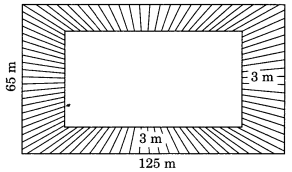Length of the park including path
= 125 m + 3m + 3m = 131 m
Breadth of the park including path
= 65m + 3m + 3m = 71m
Area of the park including path
= 131 m × 71 m = 9301 m2
∴ Area of the path
= 9301 m2 – 8125 m2 = 1176 m2
Hence, the required area = 1176 m2.

Ex 11.4 Class 7 Maths Question 3.
A picture is painted on a cardboard 8 cm long and 5 cm wide such that there is a margin of 1. 5 cm along each of its sides. Find the total area of the margin.
Solution:Length = 8 cm, breadth = 5 cm
Area of the cardboard = l × b
= 8 cm × 5 cm = 40 cm2
Width of the margin = 1.5 cm
Length of the inner cardboard
= 8 cm – 1.5 × 2 cm
= 8 cm – 3 cm = 5 cm
= 5 cm – 1.5 × 2 cm
= 5 cm – 3 cm = 2 cm
Area of the inner rectangle = l × b
= 5 cm × 2 cm = 10 cm2 Area of the margin
= 40 cm2 – 10 cm2 = 30 cm2
Hence, the required area = 30 cm2.

Ex 11.4 Class 7 Maths Question 4.
A verandah of width 2.25 m is constructed all along outside a room which is 5.5 m long and 4 m wide. Find:
(i) the area of the verandah.
(ii) the cost of cementing the floor of the verandah at the rate of ₹ 200 per m2.
Solution:Length of the room = 5.5 m
Breadth of the room = 4 m
∴ Area of the room = l × b = 5.5 m × 4 m = 22 m2
Width of the verandah = 2.25 m
Length of the room including verandah
= 5.5 m + 2 × 2.25 m = 10 m
Breadth of the room including verandah
= 4 m + 2 × 2.25 m = 8.50 m2
Area of the room including verandah = l × b
= 10 m × 8.50 m = 85 m2
(i) Area of the verandah = 85 m2 – 22 m2
= 63 m2
(ii) Cost of cementing the floor of the verandah = ₹ 63 × 200 = ₹12600

Ex 11.4 Class 7 Maths Question 5.
A path 1 m wide is built along the border and inside a square garden of side 30 m. Find:
(i) the area of the path.
(ii) the cost of planting grass in the remaining portion of the garden at the rate of ₹ 40 per m2.
Solution:Area of the square garden = (Side)2
= 30 m × 30 m = 900 m2
Length of the garden excluding the path = 30 m – 2 × 1 m = 28 m
∴ Area of the garden excluding the path = 28 m × 28 m = 784 m2
(i) Area of the path = 900 m2 – 784 m2
= 116 m2
(ii) Cost of the planting the remaining portion at the rate of ₹ 40 per m2
= ₹ 40 × 784 = ₹ 31,360

Ex 11.4 Class 7 Maths Question 6.
Two cross roads, each of width 10 m, cut a right angles through the centre of a rectangular park of length 700 m and breadth 300 m and parallel to its sides. Find the area of the roads. Also find the area of the park excluding cross roads. Give the answer in hectares.
Solution:Length of the road parallel to the length of the park = 700 m
Width of the road = 10 m
∴ Area of the road = l × b = 700 m × 10 m = 7000 m2
Length of the road parallel to the breadth of the park = 300 m
Width of the road = 10 m Area of this road = l × b = 300 m × 10 = 3000 m2
= 7000 m2 + 3000 m2 – Area of the common portion
= 10,000 m2 – 10 m × 10 m
= 10,000 m2 – 100 m2
= 9900 m2 = 0.99 ha
Area of the park = l × b
= 700 m × 300 m = 210000 m2
Area of the park excluding the roads
= 210000 m2 – 9900 m2
= 200100 m2 = 20.01 ha

Ex 11.4 Class 7 Maths Question 7.
Through a rectangular field of length 90 m and breadth 60 m, two roads are constructed which are parallel to the sides and cut each other at right angles through the centre of the fields. If the width of each road is 3 m, find
(i) the area covered by the roads.
(ii) the cost of constructing the roads at of the rate of ₹ 110 per m2.
Solution: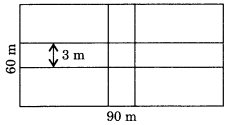Length of the road along the length of the field = 90 m
∴ Area of this road = l × b
= 90 m × 3 m = 270 m2
Similarly, the area of the road parallel to the breadth of the field = l × b
= 60 m × 3 m = 180 m2 Area of the common portion
= 3m × 3m = 9m2
(i) Area of the two roads
= 270 m2 + 180 m2 – 9 m2
= 450 m2 – 9 m2 = 441 m2
(ii) Cost of constructing the roads
= ₹ 110 × 441 = ₹ 48,510

Ex 11.4 Class 7 Maths Question 8.
Pragya wrapped a card around a circular pipe of radius 4 cm and cut off the length required of the cord. Then she wrapped it around a square box of side 4 cm (also shown). Did she have any cord left? (π = 3.14)Solution:
Length of the cord = Circumference of the circular pipe
= 2πr = 2 × 3.14 × 4 = 25.12 cm
Perimeter of the square box
= 4 × side = 4 × 4 cm = 16 cm
Length of the cord left
= 25.12 cm – 16 cm = 9.12 cm
Yes, 9.12 cm cord is left.

Ex 11.4 Class 7 Maths Question 9.
The given figure represents a rectangular lawn with a circular flower bed in the middle. Find:
(i) the area of the whole land.
(ii) the area of the flower bed.
(iii) the area of the lawn excluding the area of the flower bed.
(iv) the circumference of the flower bed.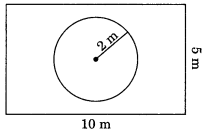Solution:
(i) Length of the lawn = 10 m
Breadth of the lawn = 5 m
Area of the lawn = l × b
= 10 m × 5 m = 50 m2
(ii) Area of the circular flower bed = πr2
$$=\frac{22}{7} \times 2 \times 2=\frac{88}{7} \mathrm{m}^{2}=12.57 \mathrm{m}^{2}$$
(iii) Area of the lawn excluding the area of the flower bed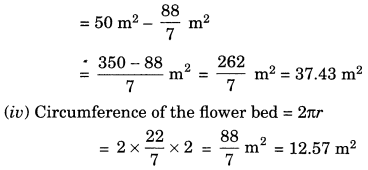Ex 11.4 Class 7 Maths Question 10.
In the following figures, find the area of the shaded portion.Solution:
(i) Area of the rectangle = l × b
= 18 cm × (6 cm + 4 cm)
= 18 cm × 10 cm = 180 cm2
Area of right triangle
$$=\frac{1}{2} \times b \times h=\frac{1}{2} \times 6 \times 10=30 \mathrm{cm}^{2}$$
Area of right ∆BCE = $$\frac{1}{2}$$ × b × h
= $$\frac{1}{2}$$ × 8 × 10 =40 cm2
Area of the two right triangles
= 30 cm2 + 40 cm2 = 70 cm2
= 180 cm2 – 70 cm2 = 110 cm2
(ii) Area of the square PQRS = (Side)2
= (20)2 = 400 cm2Area of the three triangles
= 50 cm2 + 100 cm2 + 100 cm2 = 250 cm2
= 400 cm2 – 250 cm2 = 150 cm2

Ex 11.4 Class 7 Maths Question 11.
Find the area of the quadrilateral ABCD. Here, AC = 22 cm, BM = 3 cm, DN = 3 cm, and BM ⊥ AC, DN ⊥ AC.Solution: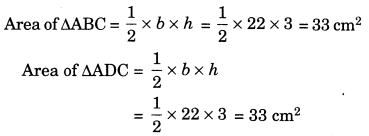= Area of ∆ABC + Area of ∆ADC
= 33 cm2 + 33 cm2 = 66 cm2
Hence, the required area = 66 cm2.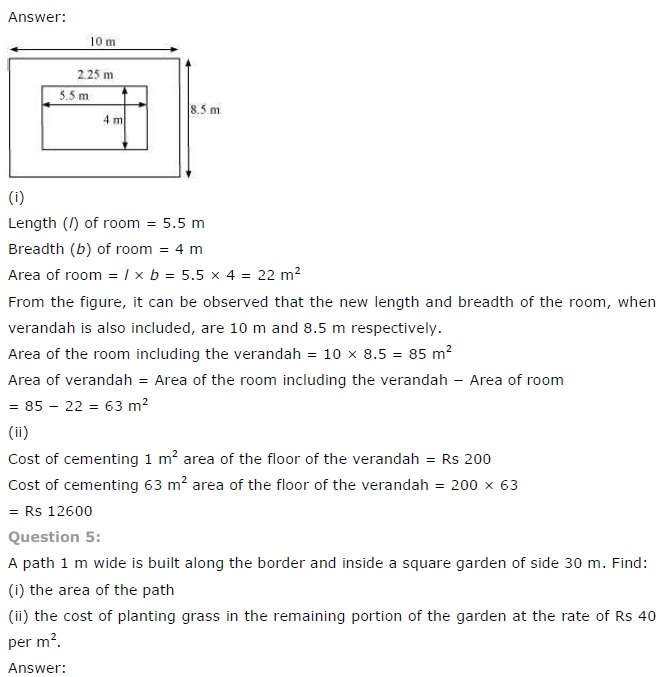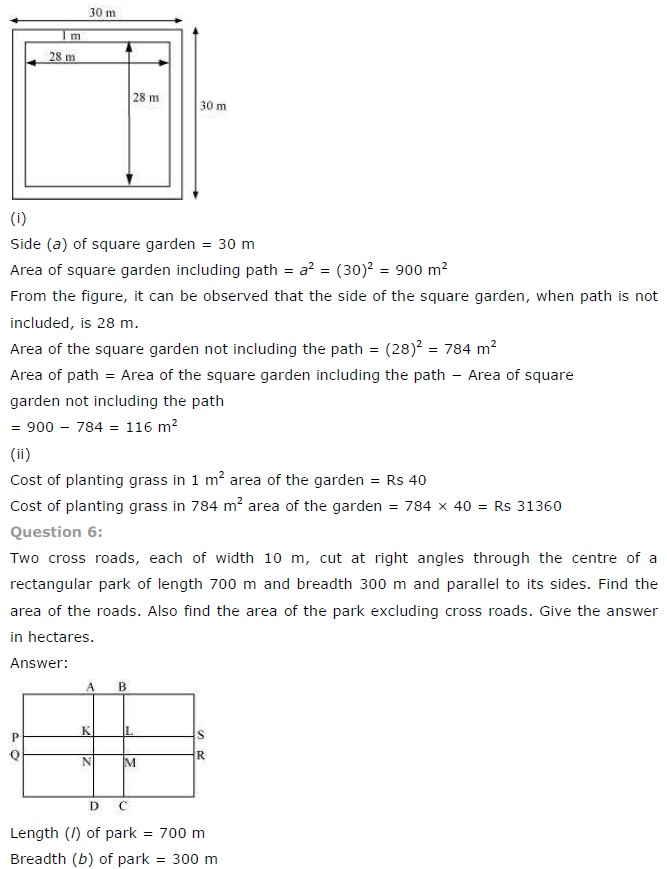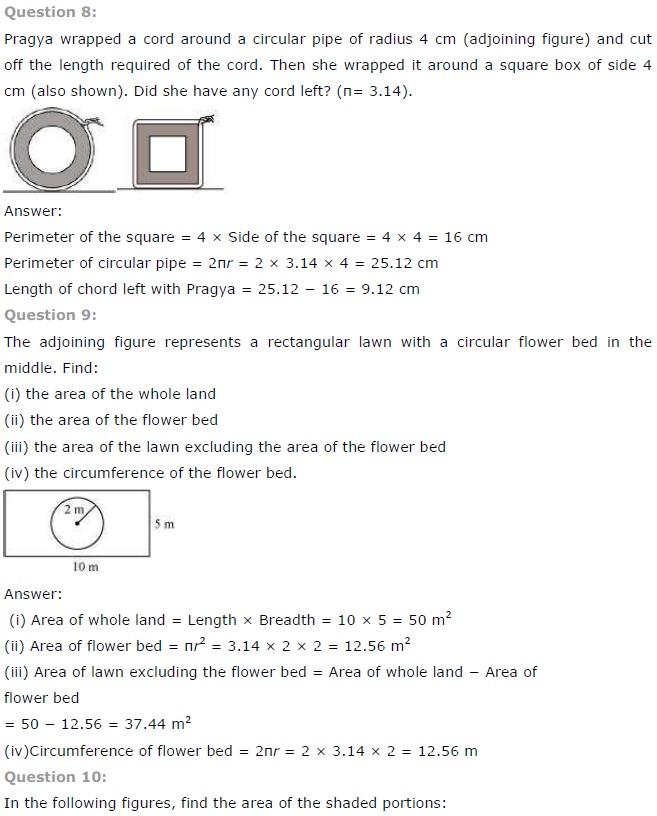+